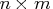Previous Page | Next Page

 Module Library

## MEDIAN Function

returns the median of numeric data

MEDIAN( matrix)

where matrix is anymatrix.

The MEDIAN function returns the median value for each column in the matrix. It computes the median of univariate numeric data contained in the specified matrix. When the number of data points is odd, it returns the middle element from the sorted order. When the number of data points is even, it returns the mean of the middle two elements. Missing values are ignored. If all values in a column are missing, the return value for that column is missing.Previous Page | Next Page | Top of Page